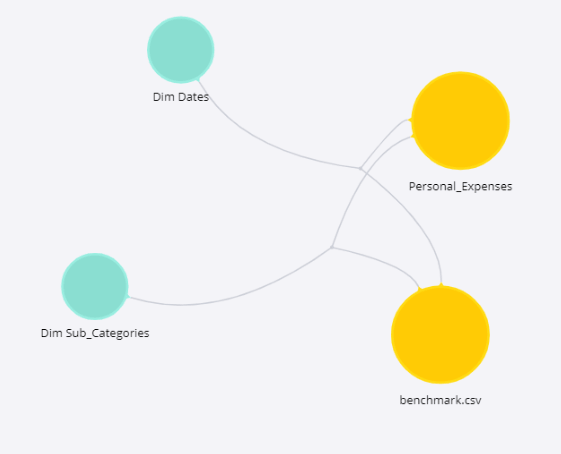High Level Design

Overview

Scorecards are used for comparing an entity to a benchmark for monitoring and measuring performance. Personal expenses are hard to keep track of due to a large number of transactions. In order for one to know in which areas it should be easier to decrease, one needs to take into consideration also the standard cost of living which is done in this case by comparing to the benchmark.

Goals

Decrease personal expenses by identifying areas that are excessively high.

Objectives

• Understand current state of expenses.
• Identify areas in which one is spending too much compared to benchmark.
• Identify areas in which one is spending less compared to benchmark.
• Identify areas in which the expense grows monthly.

KPI Architecture

 Objectives KPIs Measures Data Source 1.Understand current state of expenses. Total Expenses SUM(Amount) Personal_Expenses Expenses Distribution SUM(Amount) by Category Personal_Expenses, Dim Sub_Category Expense Amount per Category SUM(Amount) by Category Personal_Expenses, Dim Sub_Category Total Expenses Compared to Benchmark SUM(Amount)-SUM(Avg_Amount)/SUM(Amount) Personal Expenses, Benchmark 2. Identify areas in which one is spending too much compared to benchmark. Highest Expense Category Compared to Benchmark SUM(Amount)-SUM(Avg_Amount) Personal Expenses, Benchmark, Dim Sub_Category Expenses Compared to 80-120% of Benchmark per Category 0.8*SUM(Avg_Amount), SUM(Avg_Amount), 1.2*SUM(Avg_Amount), SUM(Amount) Personal Expenses, Benchmark, Dim Sub_Category 3. Identify areas in which one is spending less compared to benchmark. Lowest Expense Category Compared to Benchmark SUM(Amount)-SUM(Avg_Amount) Personal Expenses, Benchmark, Dim Sub_Category Expenses Compared to 80-120% of Benchmark per Category 0.8*SUM(Avg_Amount), SUM(Avg_Amount), 1.2*SUM(Avg_Amount), SUM(Amount) Personal Expenses, Benchmark, Dim Sub_Category 4. Identify areas in which the expense grows monthly. Monthly Growth of Expense Amount per Category GROWTHPASTMONTH(SUM(Amount)) Personal Expenses, Dim Sub_Category, Dim Dates

Entities Relationship DiagramImplementation Kit

The following resources will enable you to design your dashboard and data model with sample data and then apply it to your own data. Note that you will need to have a previously installed version of Sisense (you can use the free trial version if you’re not a customer).

TOP